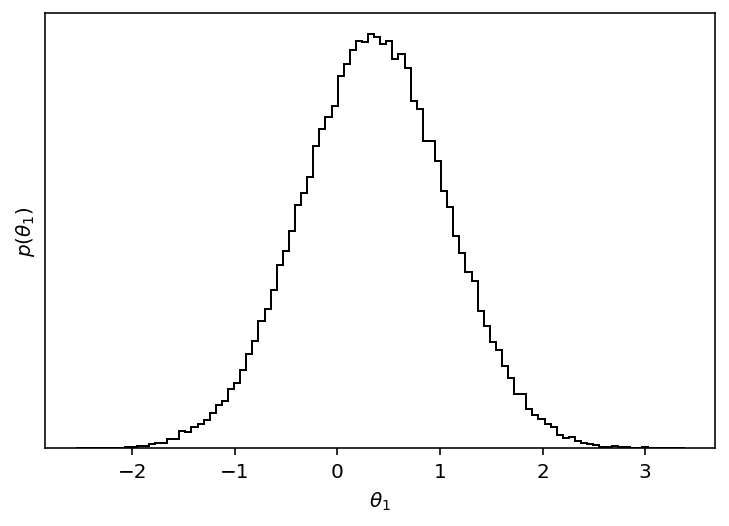# Quickstart#

Hide code cell content
%config InlineBackend.figure_format = "retina"

from matplotlib import rcParams

rcParams["savefig.dpi"] = 100
rcParams["figure.dpi"] = 100
rcParams["font.size"] = 20


The easiest way to get started with using emcee is to use it for a project. To get you started, here’s an annotated, fully-functional example that demonstrates a standard usage pattern.

## How to sample a multi-dimensional Gaussian#

We’re going to demonstrate how you might draw samples from the multivariate Gaussian density given by:

$p(\vec{x}) \propto \exp \left [ - \frac{1}{2} (\vec{x} - \vec{\mu})^\mathrm{T} \, \Sigma ^{-1} \, (\vec{x} - \vec{\mu}) \right ]$

where $$\vec{\mu}$$ is an $$N$$-dimensional vector position of the mean of the density and $$\Sigma$$ is the square N-by-N covariance matrix.

The first thing that we need to do is import the necessary modules:

import numpy as np


Then, we’ll code up a Python function that returns the density $$p(\vec{x})$$ for specific values of $$\vec{x}$$, $$\vec{\mu}$$ and $$\Sigma^{-1}$$. In fact, emcee actually requires the logarithm of $$p$$. We’ll call it log_prob:

def log_prob(x, mu, cov):
diff = x - mu
return -0.5 * np.dot(diff, np.linalg.solve(cov, diff))


It is important that the first argument of the probability function is the position of a single “walker” (a N dimensional numpy array). The following arguments are going to be constant every time the function is called and the values come from the args parameter of our EnsembleSampler that we’ll see soon.

Now, we’ll set up the specific values of those “hyperparameters” in 5 dimensions:

ndim = 5

np.random.seed(42)
means = np.random.rand(ndim)

cov = 0.5 - np.random.rand(ndim**2).reshape((ndim, ndim))
cov = np.triu(cov)
cov += cov.T - np.diag(cov.diagonal())
cov = np.dot(cov, cov)


and where cov is $$\Sigma$$.

How about we use 32 walkers? Before we go on, we need to guess a starting point for each of the 32 walkers. This position will be a 5-dimensional vector so the initial guess should be a 32-by-5 array. It’s not a very good guess but we’ll just guess a random number between 0 and 1 for each component:

nwalkers = 32
p0 = np.random.rand(nwalkers, ndim)


Now that we’ve gotten past all the bookkeeping stuff, we can move on to the fun stuff. The main interface provided by emcee is the EnsembleSampler object so let’s get ourselves one of those:

import emcee

sampler = emcee.EnsembleSampler(nwalkers, ndim, log_prob, args=[means, cov])


Remember how our function log_prob required two extra arguments when it was called? By setting up our sampler with the args argument, we’re saying that the probability function should be called as:

log_prob(p0, means, cov)

-2.596094589085444


If we didn’t provide any args parameter, the calling sequence would be log_prob(p0) instead.

It’s generally a good idea to run a few “burn-in” steps in your MCMC chain to let the walkers explore the parameter space a bit and get settled into the maximum of the density. We’ll run a burn-in of 100 steps (yep, I just made that number up… it’s hard to really know how many steps of burn-in you’ll need before you start) starting from our initial guess p0:

state = sampler.run_mcmc(p0, 100)
sampler.reset()


You’ll notice that I saved the final position of the walkers (after the 100 steps) to a variable called state. You can check out what will be contained in the other output variables by looking at the documentation for the EnsembleSampler.run_mcmc() function. The call to the EnsembleSampler.reset() method clears all of the important bookkeeping parameters in the sampler so that we get a fresh start. It also clears the current positions of the walkers so it’s a good thing that we saved them first.

Now, we can do our production run of 10000 steps:

sampler.run_mcmc(state, 10000);


The samples can be accessed using the EnsembleSampler.get_chain() method. This will return an array with the shape (10000, 32, 5) giving the parameter values for each walker at each step in the chain. Take note of that shape and make sure that you know where each of those numbers come from. You can make histograms of these samples to get an estimate of the density that you were sampling:

import matplotlib.pyplot as plt

samples = sampler.get_chain(flat=True)
plt.hist(samples[:, 0], 100, color="k", histtype="step")
plt.xlabel(r"$\theta_1$")
plt.ylabel(r"$p(\theta_1)$")
plt.gca().set_yticks([]);Another good test of whether or not the sampling went well is to check the mean acceptance fraction of the ensemble using the EnsembleSampler.acceptance_fraction() property:

print(
"Mean acceptance fraction: {0:.3f}".format(
np.mean(sampler.acceptance_fraction)
)
)

Mean acceptance fraction: 0.552


and the integrated autocorrelation time (see the Autocorrelation analysis & convergence tutorial for more details)

print(
"Mean autocorrelation time: {0:.3f} steps".format(
np.mean(sampler.get_autocorr_time())
)
)

Mean autocorrelation time: 57.112 steps